# User:Tohline/Appendix/Ramblings/RiemannB90C333

(Difference between revisions)
 Revision as of 11:09, 29 May 2020 (view source)Tohline (Talk | contribs) (→Key Physical Parameters)← Older edit Revision as of 13:59, 3 June 2020 (view source)Tohline (Talk | contribs) Newer edit → Line 70: Line 70: The subscript "EFE" on Ω and λ means that the relevant frequency is given in units that have been adopted in [[[User:Tohline/Appendix/References#EFE|EFE]]], that is, in units of $~[\pi G\rho]^{1 / 2}$.  In Figure 1a, the solid purple circular marker (where the pair of purple lines cross) identifies the location of this model in the "c/a versus b/a" diagram that appears as Figure 2 on p. 902 of [http://adsabs.harvard.edu/abs/1965ApJ...142..890C S. Chandrasekhar (1965)]; essentially the same diagram appears in §49 (p. 147) of [[[User:Tohline/Appendix/References#EFE|EFE]]]. The subscript "EFE" on Ω and λ means that the relevant frequency is given in units that have been adopted in [[[User:Tohline/Appendix/References#EFE|EFE]]], that is, in units of $~[\pi G\rho]^{1 / 2}$.  In Figure 1a, the solid purple circular marker (where the pair of purple lines cross) identifies the location of this model in the "c/a versus b/a" diagram that appears as Figure 2 on p. 902 of [http://adsabs.harvard.edu/abs/1965ApJ...142..890C S. Chandrasekhar (1965)]; essentially the same diagram appears in §49 (p. 147) of [[[User:Tohline/Appendix/References#EFE|EFE]]]. + + ==Coding Steps== + + + Here we begin with a working model of [[User:Tohline/Appendix/Ramblings/RiemannB74C692#Another_S-type_Example_b74c692|b74c692]] and use incremental changes in the COLLADA-based code to construct a working model of b90c333. + +
+
• This pair of starting models has been copied from the successful modeling of Riemann S-type ellipsoids that have (b/a, c/a) = (0.74, 0.692)
• +
+
• Inertial Frame:  [KEEP] InertialB74C692aa.dae [02 May 2020]
• +
• Rotating Frame:  [KEEP] TestMulti74.dae [02 June 2020]
• +
+
• FastRot74.dae
• +
+ + ===Best b90c333 Models=== + + The example models created for display in the Oculus Rift S are the following: +
+
1. Inertial Frame:
2. +
3. Rotating Frame:
4. +

# Another S-type Example b90c333

This chapter is an extension of the chapter we have titled, "Riemann Meets COLLADA & Oculus Rift S." In that chapter we used as our first example of a Riemann S-type ellipsoid the model with parameters, (b/a, c/a) = (0.41, 0.385). Here we construct a model with parameters, (b/a, c/a) = (0.90, 0.333). Other closely related chapters are listed below under the heading, "See Also".

## Key Physical Parameters

The model that we have chosen to use in our second successful construction of a COLLADA-based, 3D and interactive animation has the following properties; this model has been selected from Table 2 of our accompanying discussion of Riemann S-type ellipsoids: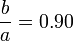$~\frac{b}{a} = 0.90$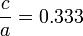$~\frac{c}{a} = 0.333$ Direct Adjoint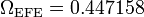$~\Omega_\mathrm{EFE} = 0.447158$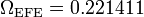$~\Omega_\mathrm{EFE} = 0.221411$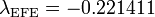$~\lambda_\mathrm{EFE} = - 0.221411$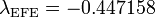$~\lambda_\mathrm{EFE} = - 0.447158$

The subscript "EFE" on Ω and λ means that the relevant frequency is given in units that have been adopted in [EFE], that is, in units of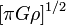$~[\pi G\rho]^{1 / 2}$. In Figure 1a, the solid purple circular marker (where the pair of purple lines cross) identifies the location of this model in the "c/a versus b/a" diagram that appears as Figure 2 on p. 902 of S. Chandrasekhar (1965); essentially the same diagram appears in §49 (p. 147) of [EFE].

## Coding Steps

Here we begin with a working model of b74c692 and use incremental changes in the COLLADA-based code to construct a working model of b90c333.

• This pair of starting models has been copied from the successful modeling of Riemann S-type ellipsoids that have (b/a, c/a) = (0.74, 0.692)
• Inertial Frame: [KEEP] InertialB74C692aa.dae [02 May 2020]
• Rotating Frame: [KEEP] TestMulti74.dae [02 June 2020]
• FastRot74.dae

### Best b90c333 Models

The example models created for display in the Oculus Rift S are the following:

1. Inertial Frame:
2. Rotating Frame: